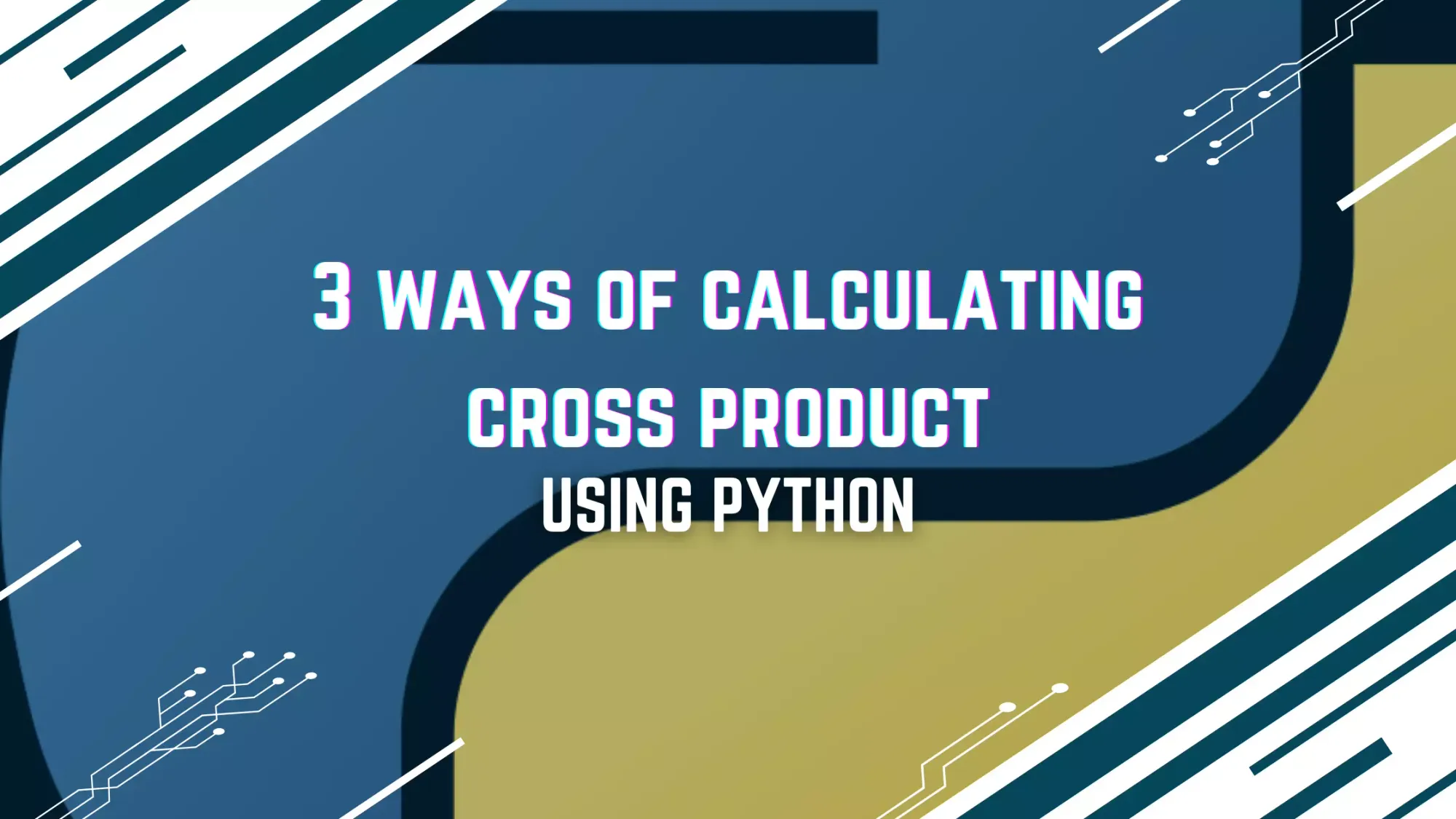# Three Ways Compute Cross Product in PythonTackling Vector Operations: Three Techniques to Compute Cross Product in Python and Their Practical Applications

Python, as a high-level and general-purpose programming language, offers a variety of libraries and built-in functionality that streamline complex computations, such as calculating the cross product of two vectors. This article will delve into three distinct methods to compute the cross product in Python using

• NumPy
• SymPy
• Pure Python implementation.

Besides, it will shed light on the practical uses and applications of the cross product in various fields.

## Definition of Cross Product

In vector calculus, the cross product (or vector product) is a binary operation on two vectors in three-dimensional space. The cross product of two vectors results in a third vector which is perpendicular to the plane containing the two original vectors.

If we denote two vectors a = [a1, a2, a3] and b = [b1, b2, b3], the cross product a x b is defined as:

$\mathbf{a} \times \mathbf{b} = (a_2b_3 - a_3b_2, a_3b_1 - a_1b_3, a_1b_2 - a_2b_1).$

This results in a vector perpendicular to both a and b with a magnitude (length) equal to the area of the parallelogram that a and b span. The direction of the resultant vector follows the right-hand rule: if you point your index finger in the direction of a and your middle finger in the direction of b, your thumb will point in the direction of a x b.

## 1. Computing Cross Product Using NumPy

NumPy, short for Numerical Python, is a pivotal Python library for handling large, multi-dimensional arrays and matrices. It provides a vast array of high-level mathematical functions to operate on these arrays.

The numpy.cross() function can be used to calculate the cross product of two vectors. Here's an example:

import numpy as np

# Define the vectors
a = np.array([1, 2, 3])
b = np.array([4, 5, 6])

# Compute the cross product
cross_product = np.cross(a, b)
print(cross_product)


In this code, numpy.array() is used to define the vectors, and numpy.cross() function calculates the cross product.

## 2. Computing Cross Product Using SymPy

SymPy is a Python library for symbolic mathematics. It aims to become a full-featured computer algebra system, while maintaining the code as simple as possible to be comprehensible and easily extensible.

To calculate the cross product with SymPy, you can use the Matrix.cross() function:

from sympy import Matrix

# Define the vectors
a = Matrix([1, 2, 3])
b = Matrix([4, 5, 6])

# Compute the cross product
cross_product = a.cross(b)
print(cross_product)


In this example, vectors are created using the Matrix() function, and the cross product is calculated using the cross() method from SymPy's Matrix class.

## 3. Manual Calculation of Cross Product

For those interested in understanding the underlying computations or in situations where external libraries may not be available, the cross product can be manually calculated. The cross product of two 3-dimensional vectors [a1, a2, a3] and [b1, b2, b3] is calculated as follows:

def cross_product(a, b):
cross_product = [a*b - a*b,
a*b - a*b,
a*b - a*b]
return cross_product

# Define the vectors
a = [1, 2, 3]
b = [4, 5, 6]

# Compute the cross product
print(cross_product(a, b))


The cross_product function takes two vectors as inputs and outputs their cross product.

## Practical Uses and Applications of Cross Product

The cross product has numerous applications across various fields, thanks to its inherent geometric interpretation.

1. Physics and Engineering: In physics, the cross product is heavily used in the study of forces and motion. For instance, it is used to calculate the torque exerted by a force (torque is the cross product of the radius vector and the force vector). In electromagnetic theory, the cross product is used in Maxwell's equations and the Lorentz force law.
2. Computer Graphics and Vision: The cross product plays a vital role in computer graphics, where it's used to determine the angle between two vectors, find perpendicular vectors, and compute normals of surfaces. In computer vision, it helps in camera positioning and 3D reconstruction.
3. Robotics: In robotics, cross products are used to manage rotations and control robotic movement.
4. Aerospace Engineering: Cross product computation is crucial in understanding the dynamics of flight, the orientation of spacecraft, and in navigation.

## Conclusion

Python offers multiple efficient and accurate methods for cross product computation. Understanding these techniques, coupled with a grasp of the practical applications of the cross product, opens a world of possibilities for problem-solving and innovation in numerous scientific and mathematical domains. Whether you're a budding programmer, a mathematician, an engineer, or a researcher, the cross product is a fundamental vector operation that's invaluable in the toolbox of computational and theoretical skills.